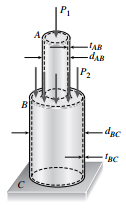# A hollow circular post ABC (see figure) supports a load P1 = 1700 lb acting at the top.

Question:

A hollow circular post ABC (see figure) supports a load P1 = 1700 lb acting at the top. A second load P2 is uniformly distributed around the cap plate at B. The diameters and thicknesses of the upper and lower parts of the post are dAB = 1.25 in., tAB = 0.5 in., dBC = 2.25 in., and tBC = 0.375 in., respectively.

(a) Calculate the normal stress σAB in the upper part of the post.

(b) If it is desired that the lower part of the post have the same compressive stress as the upper part, what should be the magnitude of the load P2?

(c) If P1 remains at 1700 lb and P2 is now set at 2260 lb, what new thickness of BC will result in the same compressive stress in both parts?